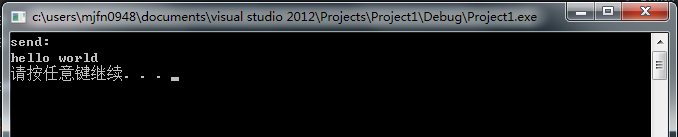# do{...}while(0)的用法,超详解

## 转载原文地址：http://blog.csdn.net/majianfei1023/article/details/45246865

#define TT_URI(want) do { \

char *ret = evhttp_uri_join(uri, url_tmp, sizeof(url_tmp)); \

tt_want(ret != NULL); \

tt_want(ret == url_tmp); \

if (strcmp(ret,want) != 0) \

TT_FAIL(("\"%s\" != \"%s\"",ret,want)); \

} while(0)



1.大家做c语言题目的时候，一道必考题就是 #define的算术运算。

#define FUNC(x) x*3+4

...

int result = 2 * FUNC(3);

result输出多少?  26？错！

1).#define M (a+b)　它的作用是指定标识符M来代替表达式(a+b)。在编写源程序时，所有的(a+b)都可由M代替，而对源程序作编译时，将先由预处理程序进行宏代换，即用(a+b)表达式去置换所有的宏名M，然后再进行编译。
2).c语言允许宏带有参数。在宏定义中的参数称为形式参数，在宏调用中的参数称为实际参数。对带参数的宏，在调用中，不仅要宏展开，而且要用实参去代换形参。(以上两句来自百度百科)

int result = 2 * x * 3 + 4
x用实参3代替就是：
int result = 2 * 3 * 3 + 4 = 22而不是26.

void print()

{

cout<<"print: "<<endl;

}

void send()

{

cout <<"send: "<<endl;

}

#define LOG print();send();

int main(){

if (false)

LOG

cout <<"hello world"<<endl;

system("pause");

return 0;

}if (false)

print();

send();

cout <<"hello world"<<endl;

#define LOG {print();send();};

if (false)

{

print();

send();

};

else

{

cout <<"hello"<<endl;

}

#define LOG do{print();send();}while (0);

int main(){

if (false)

LOG

else

{

cout <<"hello"<<endl;

}

cout <<"hello world"<<endl;

system("pause");

return 0;

}

if (false)

do{

print();

send();

}while (0);

else

{

cout <<"hello"<<endl;

}

cout <<"hello world"<<endl;



int dosomething()

{

return 0;

}

int clear()

{

}

int foo()

{

int error = dosomething();

if(error = 1)

{

goto END;

}

if(error = 2)

{

goto END;

}

END:

clear();

return 0;

}

int foo()

{

do

{

int error = dosomething();

if(error = 1)

{

break;

}

if(error = 2)

{

break;

}

} while (0);

clear();

return 0;

}